## Thursday, June 4, 2009

### The Schrödinger Equation - Corrections

[Click here for a PDF of this post with nicer formatting]
In my last post, I claimed
Additionally, we can extend from here that any quantum operator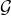is written in terms of its classical counterpart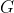by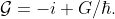Peeter Joot correctly pointed out that this result does not follow from the argument involving the Hamiltonian. While it is true that
any arbitrary unitary transformation,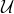, can be written as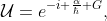whereis an Hermitian operator,
the relationship between a classicaland its quantum counterpart is not as straightforward as I claimed. In reality, we can only relate the classical Poisson brackets to the quantum mechanical commutators, and we must work from there. Perhaps I will discuss this further in a later post.

In any case, though, the derivation of the Schrödinger equation only makes use of the relationship between the classical and quantum mechanical Hamiltonians, so the remainder of the derivation still holds. I am leaving the original post up as reference, but the corrected, restructured version (with some additional, although slight, notation changes) is below.

A brief walk through classical mechanics
Say we have a function of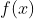and we want to translate it in space to a point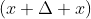, where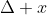need not be small. To do this, we'll find a space translation'' operator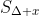which, when applied to, gives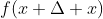. That is,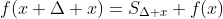(1)
We'll expandin a Taylor series: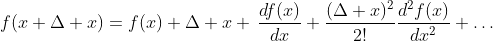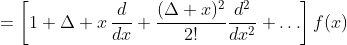(2)
which can be simplified using the series expansion of the exponential1 to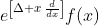(3)
from which we can conclude that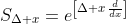(4)
If you do a similar thing with rotations around the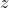-axis, you'll find that the rotation operator is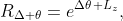(5)
where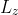is the-component of the angular momentum.

Comparing (4) and (5), we see that both have an exponential with a parameter (distance or angle) multiplied by something (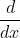or). We'll call the something the generator of the transformation.'' So, the generator of space translation isand the generator of rotation is. So, we'll write an arbitrary transformation operator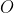through a parameter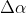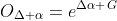(6)
whereis the generator of this particular transformation.2 See  for an example with Lorentz transformations.

From classical to quantum
Generalizing (6), we'll postulate that any arbitrary quantum mechanical (unitary) transformation operatorthrough a parametercan be written as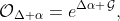(7)
whereis the quantum mechanical version of the classical operator. We'll call this the quantum mechanical generator of the transformation.'' If we have a way of relating a classical generator to a quantum mechanical one, then we have a way of finding a quantum mechanical transformation operator.

For example, in classical dynamics, the time derivative of a quantity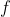is given by the Poisson bracket: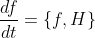(8)
where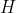is the classical Hamiltonian of the system and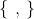is shorthand for a messy equation. In quantum mechanics this equation is replaced with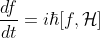(9)
where the square brackets signify a commutation relation and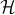is the quantum mechanical Hamiltonian. This holds true for any quantity, and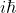is a number which commutes with everything, so we can argue that the quantum mechanical Hamiltonian operator is related to the classical Hamiltonian by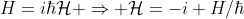(10)

Time translation of a quantum state
Consider a quantum state at timedescribed by the wavefunction. To see how the state changes with time, we want to find a time-translation'' operator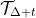which, when applied to the state, will give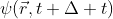. That is,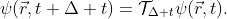(11)
From our previous discussion we know that if we know the classical generator of time translation we can writeusing (7). Classically, the generator of time translations is the Hamiltonian! So we can write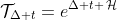(12)
where we've made the substitution from (10). Then (11) becomes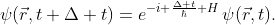(13)

This holds true for any time translation, so we'll consider a small time translation and expand (13) using a Taylor expansion3 dropping all quadratic and higher terms: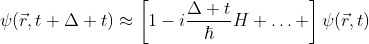(14)
Moving things around gives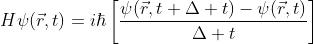(15)
In the limit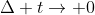the right-hand side becomes a partial derivative giving the Schrödinger equation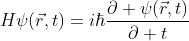(16)

For a system with conserved total energy, the classical Hamiltonian is the total energy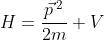(17)
which, making the substitution for quantum mechanical momentum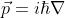and substituting into (19) gives the familiar differential equation form of the Schrödinger equation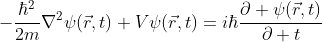(18)

References
 J.D. Jackson. Classical Electrodynamics. John Wiley & Sons, Inc., 3rd edition, 1998.
 L.D. Landau and E.M. Lifshitz. Mechanics. Pergamon Press, Oxford, UK.
 L.D. Landau and E.M. Lifshitz. Quantum Mechanics. Butterworth-Heinemann, Oxford, UK.
 H. Goldstein, C. Poole, and J. Safko. Classical Mechanics. Cambridge University Press, San Francisco, CA, 3rd edition, 2002.

1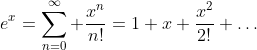2 There are other ways to do this, differing by factors ofin the definition of the generators and in the construction of the exponential, but I'm sticking with this one for now.
3 Kind of the reverse of how we got to this whole exponential notation in the first place...

#### 7 comments:

1.Hi Eli,

With the clarification made to 'From Classical to Quantum', there's a new guess in this writeup that stands out as requiring motivation (also tough). Namely, your

"making the substitution $latex \vec{p} = i \hbar \nabla$"

In Liboff, this is also pulled out of a magic hat. The only good motivation that I've seen for it was in Bohm's QT book, where it follows by Fourier transforms to switch from a position to momentum representation for the wave function. If anybody actually successfully studies from Liboff's text I imagine they also wonder where this comes from ... perhaps a topic for another blog;)

2.Peeter,
I actually think of eq. (16) as the S.E. Each step afterwards assumes a particular representation of the equation. in this post I chose the more familiar position representation. As to why, in this representation the momentum operator is the differential operator - that is a topic for another post...

3.Okay, that makes sense. It never occured to me that there ought to also be a momentum representation of (18). I'll have to play around with that, but am guessing I have to dig up my mechanics book covering Hamiltonian x,p coordinate representation first.

4.Hi Eli
I would like to thank you. I am a graduate student. I would like to ask a question: Can I learn the principles of Quantum Mechanics by myself.
Thank you again

5.Hi Anonymous,
I think you can learn the principles of QM by yourself. If you've never learned any of it before, I'd recommend starting with Griffiths' book. Peeter has taught himself most of this stuff, so you could probably ask him for advice, too.

6.I think it is hard to answer a broad question like the one posed without some idea of the starting point. I have an undergrad in engineering which provided good math fundamentals, but only basic physics. I'd rank my physics knowledge as perhaps (?) second year level undergrad physics so study of basic QM is timely (for me)

My engineering program did include a small half semester course in QM basics, and this has helped as a base for further self study. That course used the MIT book by French, which has a number of admirable features for an introductory text.

I've personally learned a lot from Bohm's book (Dover), which is cheap, and perhaps a bit old fashioned. It covers both motivational aspects and specifics with good depth. It has taken me a long time to work through that book (perhaps true of any QM text), and I am still not done.

One resource that I particulariliy liked was Cathryn Carson's History 181B: Modern Physics, on iTunesU. She is an excellent and engaging lecturer, and builds up to Quantum field theory in a descriptive and highly accessable fashion, amazingly without requiring any mathematics (it's an arts course, not a physics nor engineering). I found this gave me an excellent high level picture of why quantum theory is worth studying and how it fits in with many other aspects of physics.

7.Hello *,

I agree with Peeter, that all that is nice relationship between CM and QM, but has methodically nothing to do with a derivation of the Schrödinger equation. One methodically correct ansatz is the question, how the mechanics of an oscillator looks like, if there are no turning points? This question is admissible within Euler's representation of CM, because the basic laws/principles do not require turning points. It cannot be answered within CM, hence, it transcends CM (cf Gödel's theorem).

Best wishes,

Peter
enders@dekasges.de